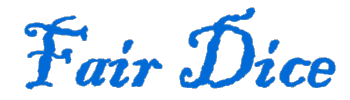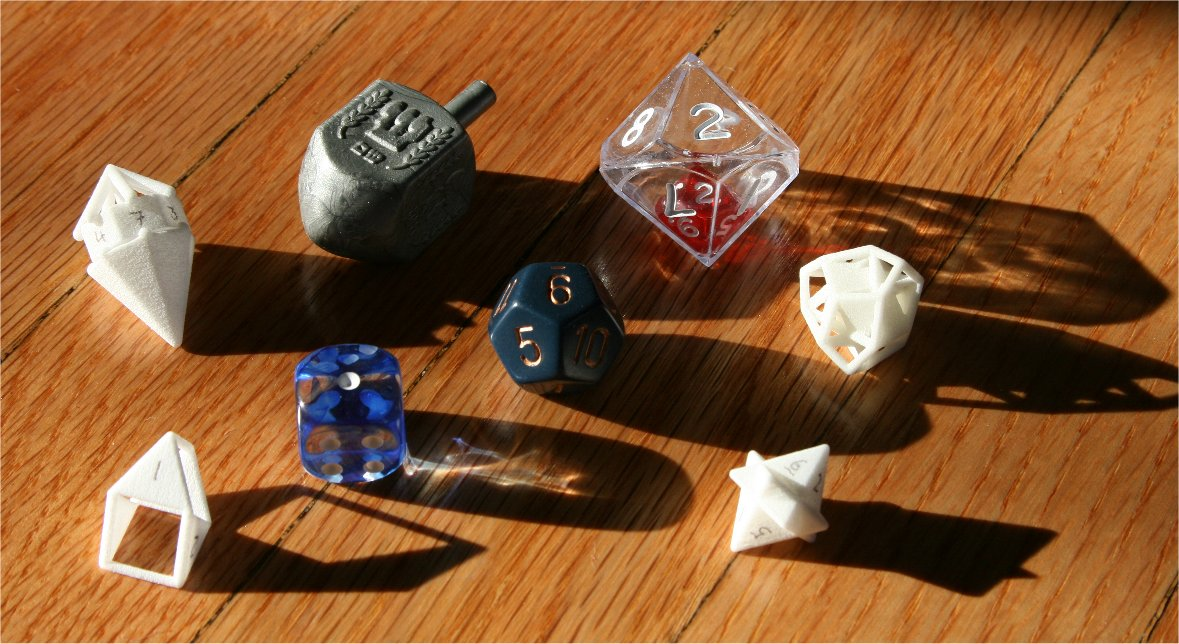Intuitively, a die is fair if each side has an equal chance of being rolled. I’ll take that a little further with the following definition.

Fair die:
A shape that is labeled such that each label has an equal probability of coming up when the shape is tossed onto a flat surface, regardless of the materials used and the angle, spin and speed with which the shape is tossed.

So which shapes make fair dice? There’s the ubiquitous 6 sided cube, of course. And anyone who has played games such as Dungeons & Dragons is familiar with dice that have 4, 6, 8, 10, 12 or 20 sides.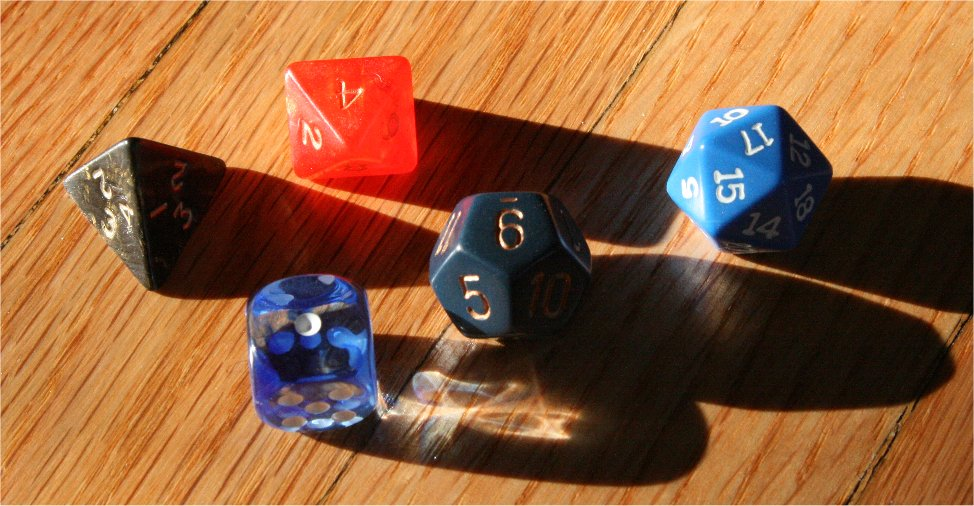But there are many other shapes that make fair dice. I categorize fair dice in the following way.

1. Every side equal
2. Sets of sides are equal
3. Symmetric modification to fair dice
4. Nested dice

### Every side equal

A polyhedron where every side is equal and has the same relationship to every other side is called an isohedron. There are 30 different classes of isohedra arranged by symmetry, most of which represent infinite families of polyhedra. The isohedra include the Platonic solids: the 4 sided tetrahedron, the 6 sided cube, the 8 sided octahedron, the 12 sided dodecahedron and the 20 sided icosahedron.

Most lists of isohedra are a bit misleading in that they show a single representative for an isohedral class without mentioning there are an infinite number of other shapes in the same symmetry class. For example, the 13 Catalan solids are all isohedra. These are very specific shapes, being duals of the Archimedean solids. However, with the exception of the two polyhedra with rhombus sides, these are all examples of infinite sets of isohedra with the same type of symmetry. My isohedra page has more examples and details on many of these shapes, while the isohedral transforms page describes how to generate them.

For more information on isohedra and fair dice, see the following references:

Some isohedra naturally make good dice. Besides the Platonic solids, there are also good dice shapes such as the rhombic dodecahedron, a 12 sided polyhedra where each side is a rhombus.

But some are a little trickier because opposite sides aren’t parallel. The tetrahedron is the most common example of this. Typical solutions include applying the same label on the tips or along the bottom edges of multiple faces so the numbers are readable when it lands on a side. If the isohedron has a vertex or edge directly opposite each side, it’s fairly straight forward to apply useful labels. But there are some isohedra where this isn’t the case, such as a triakis tetrahedron or pentagonal icositetrahedron. These shapes take a bit more work to make into usable dice. Possibilities include creating a wireframe or transparent die so the numbers can be put on a plane parallel to the down side, either on the down side itself or near the top, face up. The following picture shows how this could be done with a tetartoid.An isohedron can also be non-convex (see Isohedra with nonconvex faces for examples). My non-convex triakis tetrahedron, a 12 sided deltahedron, is a fair die. While it lands on the convex hull rather than one of the non-convex sides, the orientation of the sides works nicely in interpreting which “side” the die has landed on.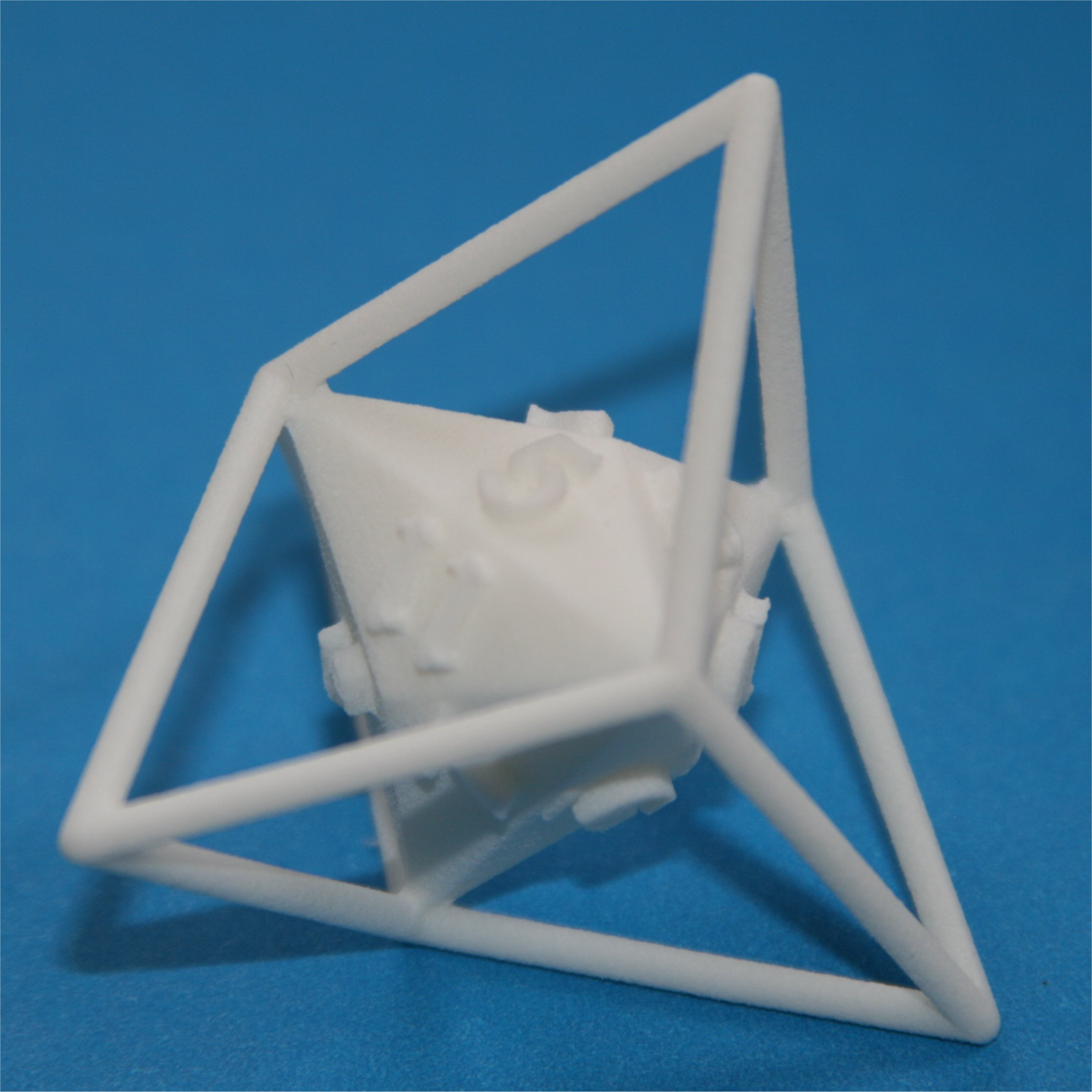Additionally, interesting fair dice can be made from isohedra with intersecting faces (e.g. my "fusion" 8 sided die derived from a trapezohedron).### Sets of sides are equal

You could number a cube 1-1-2-2-3-3 to make a fair “3 sided” die, since each label would come up with the same frequency. But more interesting are the cases where it’s not a fair die until you assign multiple sides the same number. Dice with any number of sides can be made this way. Typical examples include “barrels” with unstable ends and teetotums, which are fair dice but aren’t always recognized as such.

The simplest example is a 4 sided polyhedron made from a pair of equilateral triangles and a pair of non-equilateral isosceles triangle. This doesn’t make a fair 4 sided die since the faces aren’t equal and hence they won’t come up with equal frequency. But each of the two equilateral triangles are rolled with equal frequencey. Likewise each non-equilateral triangle comes up with equal frequency. Thus if you group the sides into two sets with one equilateral and one non-equilateral triangle per set, each of those “polysides” come up with equal frequency. This gives you a fair 2 sided die. This leads me to the following definitions.

Polyface:
A set of faces (aka polyside).
Polyisohedron:
A polyhedron where every polyface is equal and has the same relationship to every other polyface. The polyfaces are all disjoint.
Order of a polyisohedron:
The number of faces in a polyface.

All polyisohedra make fair dice. An isohedron is a polyisohedron of order 1. Note, however, that my polyisohedron definition actually has stricter requirements than a fair die. The arrangement of faces within each polyface of a polyisohedron must be congruent, but all that’s required of a fair die is that each polyside consists of the same number of each class of face. Thus I’ll define:

Polydie:
A polyhedron consisting of disjoint polysides.
Face class:
Set of faces on a polyhedron where every face is congruent and shares the same relationship with every other face on the polyhedron (e.g. all the squares in a cuboctahedron).
Fair polydie:
A polydie where each polyside consists of the same number of each class of face. Each polyside has the same probability of being rolled.

As an example, consider a Johnson solid with an entertaining name, the gyrobifastigium. It has 4 equilateral triangle faces and 4 square faces. You can pair up each triangle with a square so you have 4 polyfaces. Here’s one way to arrange them into congruent polyfaces, creating a polyisohedron: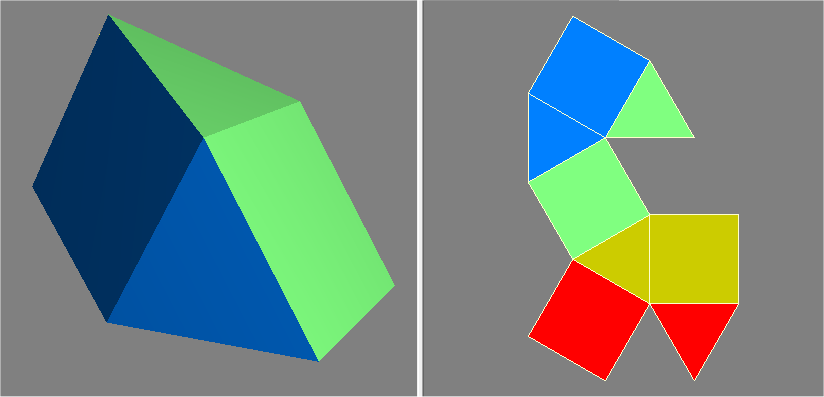This next way of pairing up the triangles and squares still makes a fair 4 polysided die, but the 4 polysides aren’t congruent, hence it’s not a polyisohedron: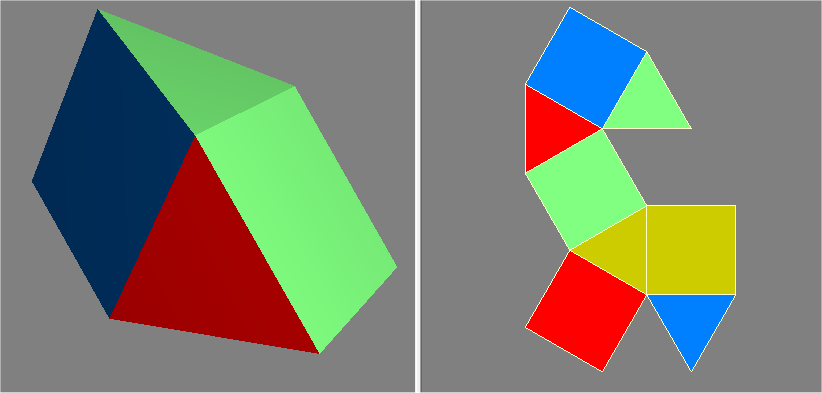Note that it’s perfectly fine if some of the faces are unstable, since all that’s required is that each corresponding face within all polyfaces shows up with equal frequency. In the gyrobifastigium shown above, the triangular faces are actually unstable, so only the squares can be landed on. Other examples include barrels with flat sides and unstable ends and dipyramids with unstable faces.

For more examples of polyisohedra, see the polyisohedra page.

### Symmetric modifications to fair dice

There are lots of ways to modify a polyisohedron while maintaining fairness, as long as those modifications are done with the proper polyisohedral symmetry.

A fair die doesn’t have to be a polyhedron, but it does have to have symmetry. Teetotums and dreidls are examples of polyisohedra that have been symmetrically modified so the unstable, unlabeled sides are rounded. Various barrels and “crystal” dice are other examples.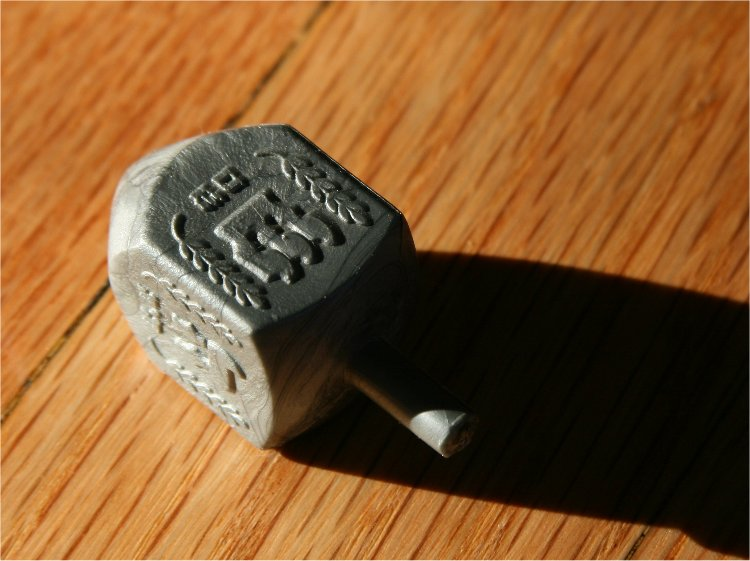Loaded dice are the canonical example of unfair dice. Each of the previous techniques for making fair dice assume that the solid (or faces) is made of a uniform material so that the weight distribution is even. However, if you add weight symmetrically, the dice are still fair. Round dice with weights distributed in a cubic arrangement are an example of this.

### Nested dice

With this technique, one die is embedded in a hollow, transparent larger die. They can be rolled and interpreted as a single unit. A good example of the benefit can be seen by trying to create a fair 100 sided die. 100 sided isohedra are shapes such as a dipyramid, unwieldy and not very graceful. The 100 sided “zocchihedron” isn’t an isohedron and hence isn’t a fair die (the sides don't appear with equal frequencey). But by nesting one 10 sided die inside another 10 sided die, you can create something that effectively has 100 sides (e.g. the outer die is used for the 10’s and the inner die is the 1’s). And since the pair is a single unit, you can easily roll multiple “100 sided dice”, unlike trying to treat pairs of icosahedra as 100 sided dice.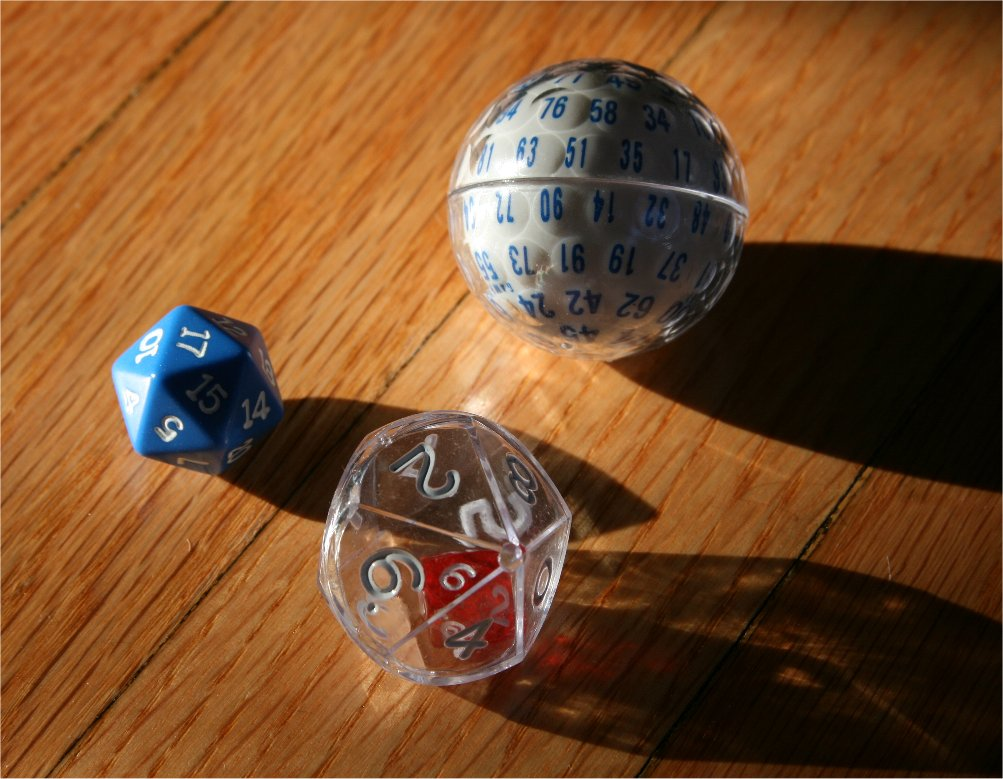This can be a nice way to achieve values that are unwieldy using a single polyisohedron such as 16 (4*4), 32 (4*8), 36 (6*6) and 100 (10*10). In theory, you could have an arbitrary number of nestings with multiple dice at each level. If one die is nested inside another, the total number of sides can be interpreted as the product of the number of sides on each individual die.

Fair dice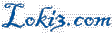© Scott Sherman 2010-2012 send comments to comments at this domain